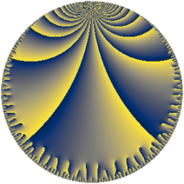# Properties

 Label 95.11.oLevel $95$ Weight $11$ Character orbit 95.o Rep. character $\chi_{95}(14,\cdot)$ Character field $\Q(\zeta_{18})$ Dimension $588$ Newform subspaces $1$ Sturm bound $110$ Trace bound $0$

# Related objects

## Defining parameters

 Level: $$N$$ $$=$$ $$95 = 5 \cdot 19$$ Weight: $$k$$ $$=$$ $$11$$ Character orbit: $$[\chi]$$ $$=$$ 95.o (of order $$18$$ and degree $$6$$) Character conductor: $$\operatorname{cond}(\chi)$$ $$=$$ $$95$$ Character field: $$\Q(\zeta_{18})$$ Newform subspaces: $$1$$ Sturm bound: $$110$$ Trace bound: $$0$$

## Dimensions

The following table gives the dimensions of various subspaces of $$M_{11}(95, [\chi])$$.

Total New Old
Modular forms 612 612 0
Cusp forms 588 588 0
Eisenstein series 24 24 0

## Trace form

 $$588 q - 1842 q^{4} - 6 q^{5} - 7302 q^{6} - 12 q^{9} + O(q^{10})$$ $$588 q - 1842 q^{4} - 6 q^{5} - 7302 q^{6} - 12 q^{9} + 314787 q^{10} + 343644 q^{11} - 1769646 q^{14} + 2373333 q^{15} + 6815562 q^{16} + 12524508 q^{19} - 5795274 q^{20} + 3990534 q^{21} - 48233604 q^{24} + 54962148 q^{25} + 159714 q^{26} - 51260712 q^{29} - 73197330 q^{30} - 18 q^{31} - 142070532 q^{34} - 153871836 q^{35} - 143737566 q^{36} + 249898896 q^{39} - 162362148 q^{40} + 329731368 q^{41} + 43247604 q^{44} - 343666425 q^{45} - 2934201618 q^{46} + 11044492350 q^{49} - 5706705726 q^{50} - 479014572 q^{51} - 3435679536 q^{54} + 2281950426 q^{55} + 4872445248 q^{59} - 3353868486 q^{60} - 1563443832 q^{61} - 38093728782 q^{64} + 2760985836 q^{65} + 9000466164 q^{66} + 7642077822 q^{69} + 18561088251 q^{70} - 15771730512 q^{71} + 13695176790 q^{74} - 8678922192 q^{76} + 15824124468 q^{79} + 11740810599 q^{80} - 3403661598 q^{81} - 109354282758 q^{84} - 3017143236 q^{85} + 1995559458 q^{86} + 37596576408 q^{89} + 21441348066 q^{90} + 51398917794 q^{91} + 18379983615 q^{95} - 166641665256 q^{96} - 138152381466 q^{99} + O(q^{100})$$

## Decomposition of $$S_{11}^{\mathrm{new}}(95, [\chi])$$ into newform subspaces

Label Dim $A$ Field CM Traces $q$-expansion
$a_{2}$ $a_{3}$ $a_{5}$ $a_{7}$
95.11.o.a $588$ $60.359$ None $$0$$ $$0$$ $$-6$$ $$0$$